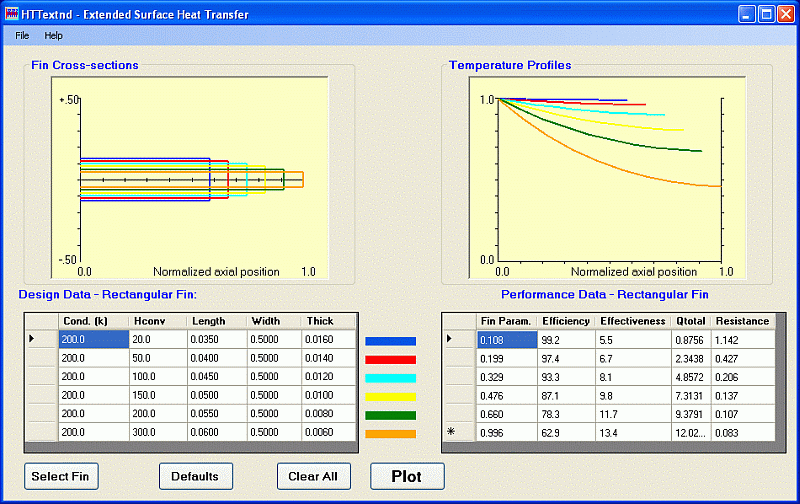# Analysis of Extended Surfaces (Fins and Heat Sinks)

UPDATED: 08/13/2022

The normal textbook treatment of heat transfer from fins involves the solution by analytical means of an ordinary differential equation describing transport by conduction along the fin and convection from its surface. Even with a straight, rectangular fin, there is the quandary about what boundary condition to apply at the tip (which is usually resolved using an “extended length”), and the solution is expressed in terms of hyperbolic functions. In the case of straight fins of triangular cross-section and annular fins of constant thickness, the solutions are given in terms of Bessel functions, which the student may or may not have studied. Also, in the latter cases they never see the temperature distribution along the fin; only an overall parameter, the fin efficiency, is usually graphed.   The major application of extended surface heat transfer is in arrays of fins (heat sinks.)

Module Description

In this module a finite-volume solution of the governing heat balance equation is used instead. Terms representing conduction into and out of a short representative segment of the fin and convection from its surface are included and the necessary derivatives of temperature in the conduction terms are expressed in terms of changes over small increments. The resulting tridiagonal system of equations is solved using publicly available software and the student has the complete temperature distribution at their disposal immediately. With that plot a good or poor fin design is readily apparent and in the latter case, may be rectified quickly. Since the algorithm does not require the evaluation of different functions for each distinct geometry, but only input of the appropriate areas for axial conduction and for convection to the fluid, this solution may be easily carried out for virtually any one-dimensional geometry.  A spreadsheet that shows clearly the procedures for using the finite-volume method on the fin equation (as implemented in HTTextnd) may be downloaded here.Interface for Extended Surface (Fin) Heat Transfer. Parameters are input in the data grid at the bottom left and the fins being analyzed are depicted in the left window. The predicted temperature distributions are returned in the right window. The fin efficiency and effectiveness are returned at the bottom right.

The user selects the fin type (straight rectangular, cylindrical pin, straight annular or triangular) and a schematic of that geometry pops up so that he or she can see how the requisite dimensions are defined. More general two-dimensional, spine and annular fins having a cross-section that may be described by a simple polynomial are also handled readily. For all geometries the user may input the thermal conductivity of the material and the surface convection coefficient for as many as six cases simultaneously. A scaled cross-sectional profile of each fin is plotted in the left window, while the resulting 1-D temperature distribution along the fins is plotted in the right window. Numerical values of the fin efficiency (η), effectiveness (ε), total heat transferred, and thermal resistance are reported in tabular form. This module also provides an input sheet so that data from a simple accompanying desktop experiment may be plotted along with the numerical predictions. Photos of a number of extended surface heat transfer applications may be accessed from the module.

Virtual Laboratory

A virtual laboratory by Jorge Navalho based on the HTTextnd module can be found here.